Contents >> Applied Mathematics >> Mathematical Statistics >> Elements of Mathematical Statistics >> Examples

Elements of math statistics - Examples

Examples 

E x a m p l e 1. Trains of underground go on a regular basis with an interval 2 minutes. Passenger leaves on platform during the casual moment of time which in any way has been not connected with the schedule. A random variable – time Т during which it should wait for a train. Find density function, mathematical expectation, dispersion, average square-law deviation and probability of what to wait to the passenger it is necessary no more half-minute.

S o l u t i o n . Density function f ( x ) =1/2 (0 < x < 2) is shown on Fig. 9.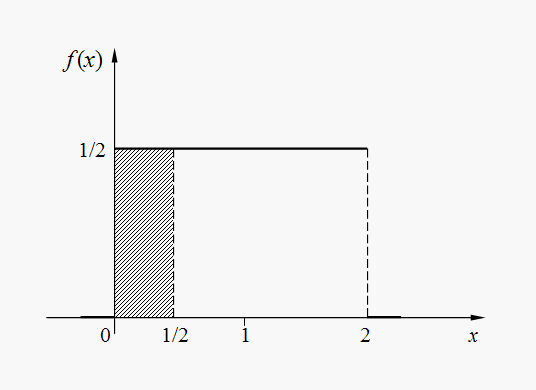Fig . 9. Density function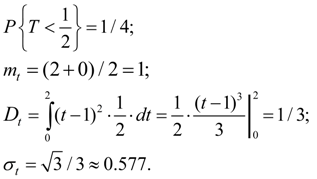E x a m p l e  2. Random variable X has exponent distribution with parameter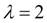. Find probability of event {1 < X < 2}.

S o l u t i o n . We have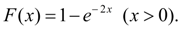The probability of hit in an interval (1, 2) is equal to an increment of function of distribution on this interval: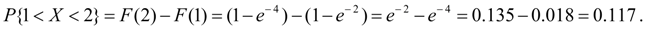E x a m p l e  3. The matrix of distribution of system of two random variables X and Y is given by the table:

 y j x i 0 2 5 1 0.1 0 0.2 2 0 0.3 0 4 0.1 0.3 0

Find numerical characteristics of system of random variables ( X , Y ): mathematical expectations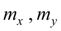, dispersions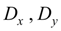, average square-law deviations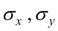, covariance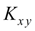and coefficient of correlation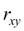.

S o l u t i o n . First we’ll find series of distribution of separate values entering into the system. Summarizing probabilities p ij , being in the 1-st, the 2-nd and the 3-rd lines, we’ll receive: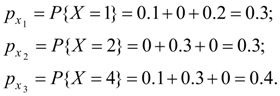Series of distribution of random variable X looks like:

 1 2 4 0.3 0.3 0.4

Mathematical expectation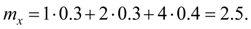From formula (8) dispersion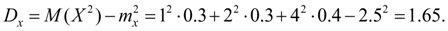Average square-law deviation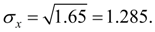Similarly summarizing probabilities p ij , being in the 1-st, the 2-nd and in the 3-rd columns, we’ll receive: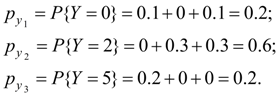Series of distribution of random variable Y looks like:

 0 2 5 0.2 0.6 0.2

Mathematical expectation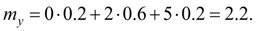From formula (8) dispersion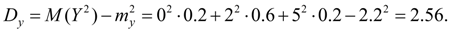Average square-law deviation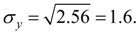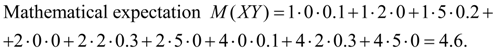Covariance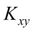from formula (52) is equal to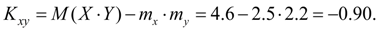Coefficient of correlation: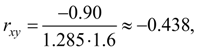thus, between random variables X and Y there is a negative linear correlation, i.e. at increase in one of them another has decreases.

 < Previous Contents Next >